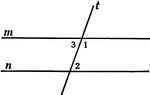### 2 Lines Cut By A Transversal

Illustration of two straight lines, m and n, cut by a transversal t.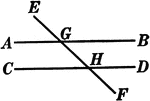### 2 Lines Cut By A Transversal

Illustration of two straight lines, AB and CD, cut by a transversal EF.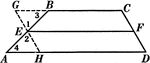### Line Bisecting Trapezoid Sides

Illustration used to prove the theorem "The line bisecting one of the non parallel sides of a trapezoid…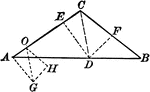### Triangle With Segments

Triangle with segments drawn.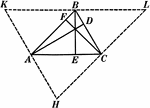### Altitudes In A Triangle

Illustration used to show "The altitudes of a triangle are concurrent."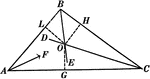### Angle Bisectors In A Triangle

Illustration used to prove "The bisectors of the angles of a triangle are concurrent in a point which…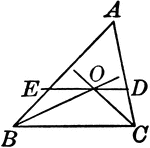### Triangle With Bisectors of Base Angles

Illustration to show if the bisector of the base angles of a triangle are drawn, and through their point…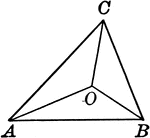### Triangle With Lines From the Vertices That Join a Point Within

Illustration to show lines drawn from the vertices to join at a point within the triangle.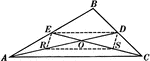### Medians In A Triangle

Illustration used to show "Any two medians of a triangle intersect each other in a trisection point…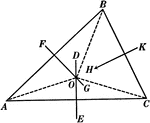### Perpendicular Bisectors In A Triangle

Illustration used to prove "The perpendicular bisectors of the sides of a triangle are concurrent in…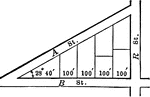### Triangular City Block With Angles and Lengths

Illustration showing an angle of 23 degrees 40 minutes making a triangle in a city block and marking…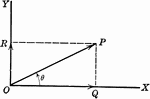### Vector

OP is a line representing a vector (directed quantity)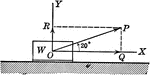### Vector Showing Force at Angle of 20 degrees

OP is a vector (directed quantity) showing the force by a weight acting on it at an angle of 20 degrees.### Line, Vertical

A vertical line with arrows at both ends to show that it extends indefinitely.### Line, Vertical

A vertical line with arrows at both ends to show that it extends indefinitely.### Drawing a Vertical Line

Vertical lines are drawn by using a triangle held against the T-square.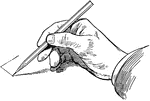### Sketching Vertical Line

Vertical line is drawn downward with the pencil held with freedom, not close to the point.